# Tuesday August 22nd

## Nanodegree

• We’re doing fun stuff like this in Python!

## A movie site that shows images of movies you like, and gives descriptions## Click on the image and it will play the trailer!• Learning about classes and objects, and how to instantiate so that they call on different objects.

## Teaching myself Mathematica

• In the midst of my Nanodegree and before my C++ class continues, I thought that I should use a 15-day trial of Mathematica.

## Wait..what is Mathematica?

• It’s a tool used for numerical computation, data visualization, primarily used by mathematicians, scientists and engineers. It is known for its precision and was invented by Stephen Wolfram.

• Here is the wiki

## But why?

• Here is a great article on who uses Mathematica.

• There is also quite an active Mathematica Stack Exchange. Do subscribe!

• It’s always been something I’ve been interested in learning a bit of; I can’t put my finger on why right now.

• That being said, I did finish a project early, and I only have a 15-day trial, so I thought that this would be perfect timing.

## So…

• My beginnings were from this tutorial

• I liked it so much, I bought a book on it, and plan on continuing, taking baby steps to learn.

## It’s like Matlab, LaTeX and Jupyter had a baby!

• Seriously, if you’re familiar with those, things will seem very familiar! It’s super fun!

• I’m still learning, but here’s some of what I learned today!

## Defining and getting used to Syntax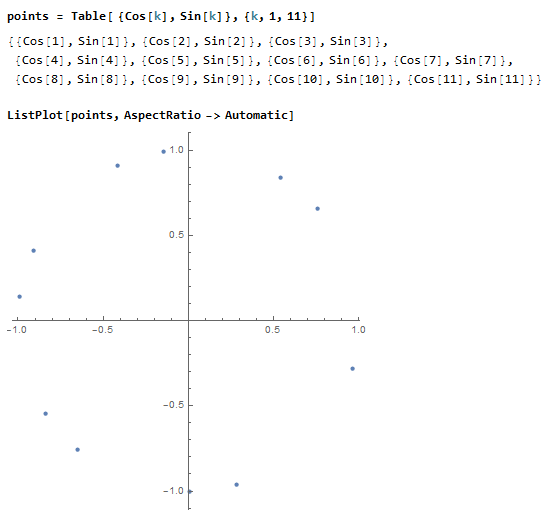## Algebra using power and subscripting (very similar to LaTeX)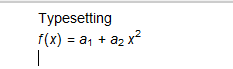\

## More typesetting

• Whoops, I forgot a bracket!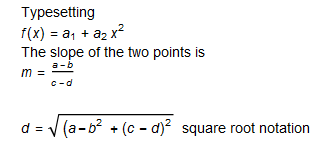## Precision in calculations (this is with 30 digits of precision)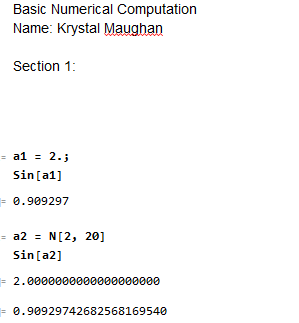## One-based indexing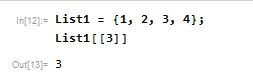## Appending to a list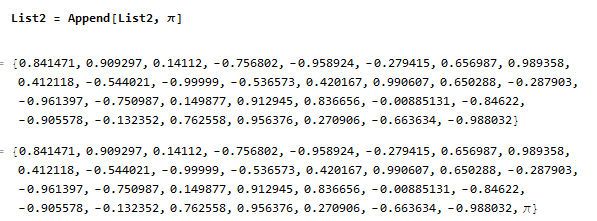## Appended List with pi and finding the Length of a List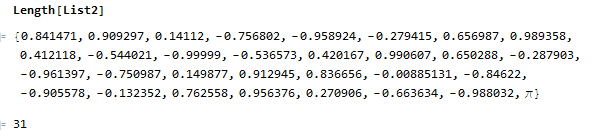## Substitution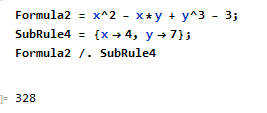## Plotting a function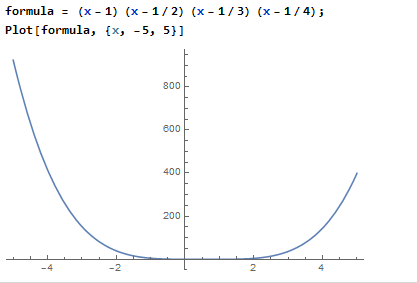## Defining the Plot Range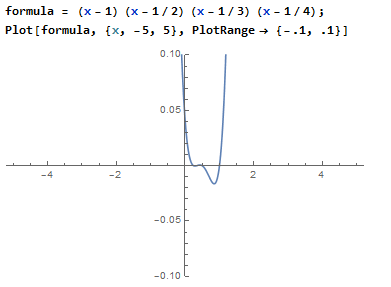## Adding Details to the Plot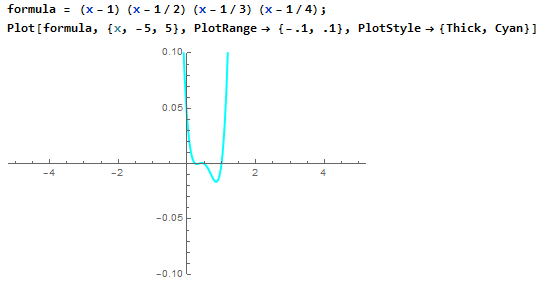## Functions Learned

``````Alt+7 -> Enter mode
Ctrl + ( -> Math mode
Ctrl + _ -> subscript
Ctrl + space -> out of subscript mode
Ctrl + ^ -> power
Ctrl + / -> (fractions) and arrow down for denominator
Ctrl + 2 -> square root
Alt + 7 -> type words (not mathematical commands)
Shift + Enter -> Evaluate
Alt + . -> Abort Computation

a2 = N[2, 20] -> 2 means 2 is numerical
and 20 is accuracy (ie 20 decimals)

------------------------------

one-based indexing
Length[Listname] -> gives length of list

---------
Plotting
---------
functiontoplot = x + 5
Plot[function, {x, value, value}, PlotRange->
{range1, range2}, PlotStyle->{Thick,Color}]

Two figures overlapping -> Show[Figure1, Figure2]

``````

## Things to do

• Continue with Nanodegree (finish up lessons and get to Project by end of this week..hopefully)
• Finish Application (probably will be done by Wednesday)
• Continue with Mathematica tutorials and experimentation (when you obtain book, read and work through)

## Katas

• Create a pattern that looks like
``````1
22
333
4444
55555
``````
• My solution
``````def pattern(n):
arr = ""
if n < 1:
return ""
elif n == 1:
return "1"
else:
for i in range(2, n+1):
arr =  arr + "\n" + str(i) * (i)
return "1" + arr
``````
• Find first non-consecutive number
``````def first_non_consecutive(arr):
arr1 = []
arr2 = []
a = arr
b = arr[-1]

# find full list with no elements left out
for i in range(a, b+1):
arr1.append(i)

# find list difference between two lists
d = list(set(arr1) - set(arr))
e = sorted(d)
# value is one more than the difference
try:
return e+1
# catch values where the lists are the same
except:
return None
``````
Written on August 22, 2017